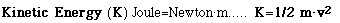Hands-On-Physics

Heat Energy (Q)

Heat energy can be put into an object, warming it from temperature T1 to temperature T2. The increase in heat (Q) is proportional to the temperature change (T2 - T1) and proportional to the mass (m) of the object being warmed. The proportionality constant (c), called the specific heat, depends on the material the object is made of. Heat change

Q = c * m * (T2 - T1)

Heat is a form of energy, so it has the units of energy. In the SI system, this unit is the Joule . Many other units to measure thermal energy are in common use. Calories and BTU's are common heat units.Notice that this energy of motion is proportional to the square of the speed. The unit of Joule may also be expressed as kg(m/sec)(m/sec).

A | B | C | D | E | F | G | H | I | J | K | L | M | N
O | P | Q | R | S | T | U | V | W | X | Y | Z
Top of Glossary